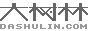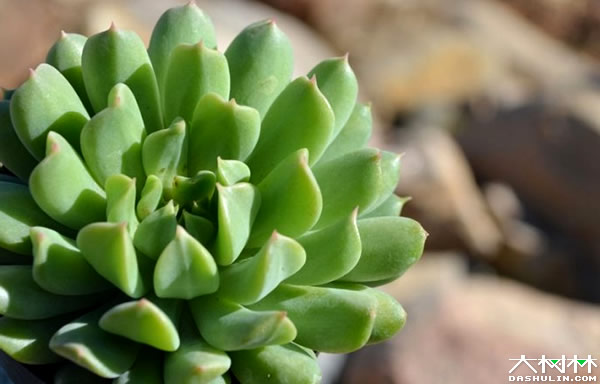﻿ 景天科多肉杂交公式 | 大树林植物网# 景天科多肉杂交公式一般来说，母本在前，父本在后。按照规律，杂交公式中得出的品种是优选品种。也就是说苯巴蒂斯是静夜X大和锦的优选，不一定静夜×大和锦后种荚里都是苯巴的种子，存在一定的概率，请注意！
另外大家一般说起的大和锦，其实已经是大和锦的杂交品种了，所以想出苯巴 赫拉 央金之类的，还需先买原始的大和锦。

• 静夜×大和锦=苯巴蒂斯
• 静夜×胧月=白牡丹
• 静夜×花月夜=月光女神
• 静夜×丽娜莲=露娜莲
• 静夜×鲁氏=克拉拉
• 静夜×特玉莲=爱尔兰薄荷
• 静夜×花司=戴伦
• 静夜×锦司晃=玫瑰莲
• 静夜×粉蔓=船长甘草
• 静夜×月影=静月
• 静夜×吉娃娃=Tippy
• 静夜×乙女心=柳叶莲华
• 静夜×多茎莲=红化妆
• 静夜×黑爪=红爪
• 大和锦×桃之卵=葡萄
• 桃之卵×卡罗拉=奥普
• 大和锦×静夜=法比奥拉
• 大和锦×E.”Big Red”=央金
• 大和锦（杂）×晚霞=赫拉
• 姬莲×蓝石莲=蓝姬莲
• 玉蝶×姬莲=娜娜胡可
• 黑爪×姬莲=野蔷薇精灵
• 小和锦×姬莲=皇冠
• 广寒宫 × 沙维娜=晨光
• 广寒宫 × 沙维娜=晨星
• 广寒宫 × 沙维娜=晚霞
• 广寒宫 × 鲁氏=多明戈
• 广寒宫 × 鲁氏=赫斯塔
• 广寒宫 × 多明戈=星期天
• 广寒宫 × 皮氏=蓝鸟
• 广寒宫 × 星美人=霜之朝
• 沙维娜 × 广寒宫=Pinky
• 雪莲 × 林赛=芙蓉雪莲
• 雪莲 × 卡罗拉=梦露
• 雪莲 × 特玉莲=特玉雪莲
• 古紫 × 沙维娜=黑王子
• 月影 × 粉彩莲=紫珍珠
• 月影 × 静夜=月静
• 花月夜 × 厚叶月影=月亮仙子
• 花月夜 × 大和锦=猎户座
• 花月夜 × 霜之鹤=花之鹤
• 锦晃星× 锦司晃=白闪冠
• 特玉莲× 黛比=丘比特
• 胧月 × 乙女心=秋丽
• 胧月 × 冬云系如贝拉=厚叶旭鹤
• 胧月 ×  珊瑚珠=姬胧月
• 雪莲 × 霜之鹤=白凤﻿
*必填
*必填